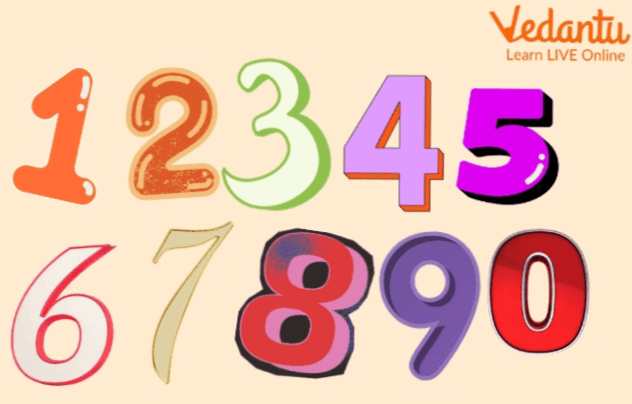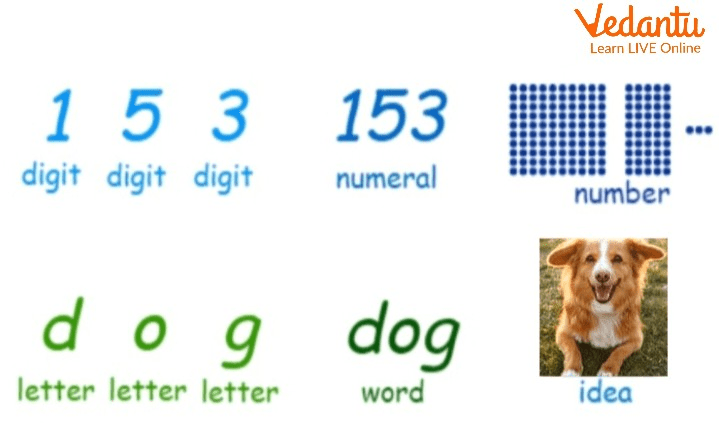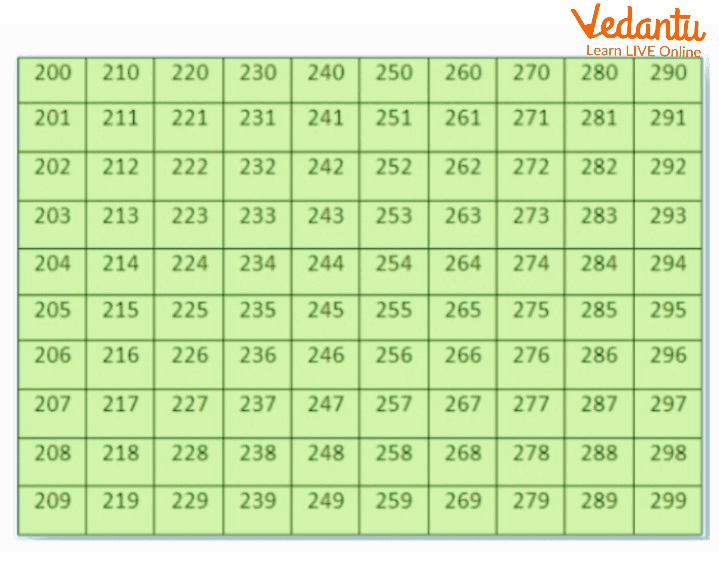Courses
Courses for Kids
Free study material
Free LIVE classes
More## 200 Numbers Group

We are going to learn about numbers by taking numbers from 200 to 299. The natural numbers that may divide the initial number (200) evenly are known as factors of 200 in mathematics. These elements are also known as 200 divisors. Given that 200 is an even composite number, there will be more than two elements. Another way to define factors of 200 is to say that they exist when the sum of two natural numbers is equal to 200. We may utilize straightforward division techniques and prime factorization techniques to identify these components. And after that, we classify these groups of numbers into even or odd.Numbers in Mathematics

## What is Numeral?

Any figure or symbol, or group of figures or symbols representing a number is known as a numeral.Numerals

## Numbers From 200 to 209

When 1 is added to 199, the sum is 200 (two hundred). When we go on increasing 1 in every number after 200, we get 201, 202, 203, 204, 205, 206, 207, 208, 209, 210, ………….., 219, 220, 221, …………….., 231, 232, ……………., 245, 246, …………….., 250, 251, ……………., 260, …………….., 270, …………….., 280, 281, ………………, 290, ……………., 298, 299.

If we put 1, 2, 3, 4, 5, 6, 7, 8, and 9 respectively at one’s place instead of 0 in 200, we get 201, 202, 203, 204, 205, 206, 207, 208, 209.

Now if we put two-digit numbers 10, 11, 12, 13, 14, 15, 16, 17, ……………, 27, 28, 29, ……………, 40, 41, ……………, 58, 59, …………….., 70, ………………., 83, 84, ……………, 91, ……………. to 99 after 2 instead of 00 in 200, we get 210, 211, 212, ………….., 220, 221, ……………., 230, ………………., 241, 242, ……………, 250, …………….., 260, …………….., 270, 271, …………….., 280, ………………, 298, 299.

Thus, we get all the numbers from 200 to 299, which are given in the following table.Numbers From 200 to 299

We see the following specialties in the numerals from 200 to 299:

1. The numerals from 200 to 299 have the digit 2 in their hundred’s place. We see in each column and in each row, the digit in the hundred’s place is the same i.e., 2.

2. In each column, the digit in the ten’s place is the same, i.e., 0, 1, 2, 3, 4, 5, 6, 7, 8, and 9 respectively. After every ten numbers, i.e., 200 to 209, 210 to 219, 220 to 229, 230 to 239, 240 to 249, 250 to 259, 260 to 269, 270 to 279, 280 to 289, and 290 to 299, the digit in ten’s place is changed.

3. In each column, the digits in one’s place are in ascending order from 0 to 9. Thus, each succeeding number is increased by 1.

4. In each row, each succeeding number increases by 10, i.e., 200, 210, 220, 230, 240, 250, 260, 270, 280, 290.

5. The numbers from 200 to 299 are formed by placing 00, 01, 02, 03, 04, 05, 06, 07, 08, 09, 11, …………….., 21, 22, ………………, 30, ………………, 39, 40, ………………., 51, …………….60, ………….., 70, 71, …………….., 80, …………….., 90, ……………, 98, 99 respectively after 2. The numbers from 00, 01 to 99 are placed after 2.

## How to Find Groups of Ten in any Numbers?

For finding groups of ten, it is necessary for a number to be equal to or greater than 10 then we only able to find groups of ten on that number.

To find groups of ten you need to divide the number by 10 and the quotient of that division is known as groups of ten Groups of ten of a number are defined as how many 10 are present in that number. E.g.

1. 199 means 19 groups of ten and 9 more.

2. 200 means 20 groups of ten.

3. 350 means 35 groups of ten.Group of 10 Each.

In the above image, we can see that each box contains 10 quantities of different items. When we add all groups of 10 items it will become a total of 100 items.

## Even Numbers

Even numbers are that type of numbers that are completely divisible by 2 meaning on division 2 that number leaves 0 remainders.

E.g 2, 10,200,2900… etc

## Odd Numbers

Numbers that when divided by 2 leaves remainder(1) or you can say that the numbers which are not completely divisible by 2 are knowns odd numbers

E.g 1,3,5,11… etc

## Even Numbers 1 to 200

There are a total of 100 even numbers and 100 odd numbers between 1 to 200, now it is your task to find which number is even and which is odd. You can also use a pattern as you know that 2 is the smallest even number so just add 2 to 2 and the sum is also even, so if we add 2 to the even number the sum that comes is even the same goes for odd if we add 2 to the odd number their sum is also odd.

## Solved Examples

1. How many groups of 10 in 230?

Ans: 23, on dividing 230 by 10 we get 23.

2. What comes after 199?

Ans: 200, we add 1 to 199 and the result of that is our answer

3. How to Write Numbers in Words 100 to 200?

Ans: We adhere to a set of criteria while writing the names of numbers from 100 to 200. The above number is expressed in its extended form as follows: 198 = 100 + 98, where the ones and tens place digits are treated as a single unit. Then, using the usual criteria, write and combine the individual names of all the addends as shown below: 100 + 98 = one hundred ninety-eight.

## Conclusion

In this article we learned about numbers and numerals also with that we learned to find groups of ten in any number which is greater than 10 that also understand the difference between even and odd numbers.

Last updated date: 21st Sep 2023
Total views: 79.5k
Views today: 1.79k

## FAQs on Learning About Numbers

1. Which is the smallest odd number?

1 is the smallest odd number.

2. What is a table of 200?

The multiplication table known as the "Table of 200" shows the result of multiplying 200 by a series of successive natural integers.

3. Is 0 an even number or an odd number?

0 is an even number, subtract 2 from 2 you will get 0.

4. Which is the smallest even number?

0, is the smallest even number.